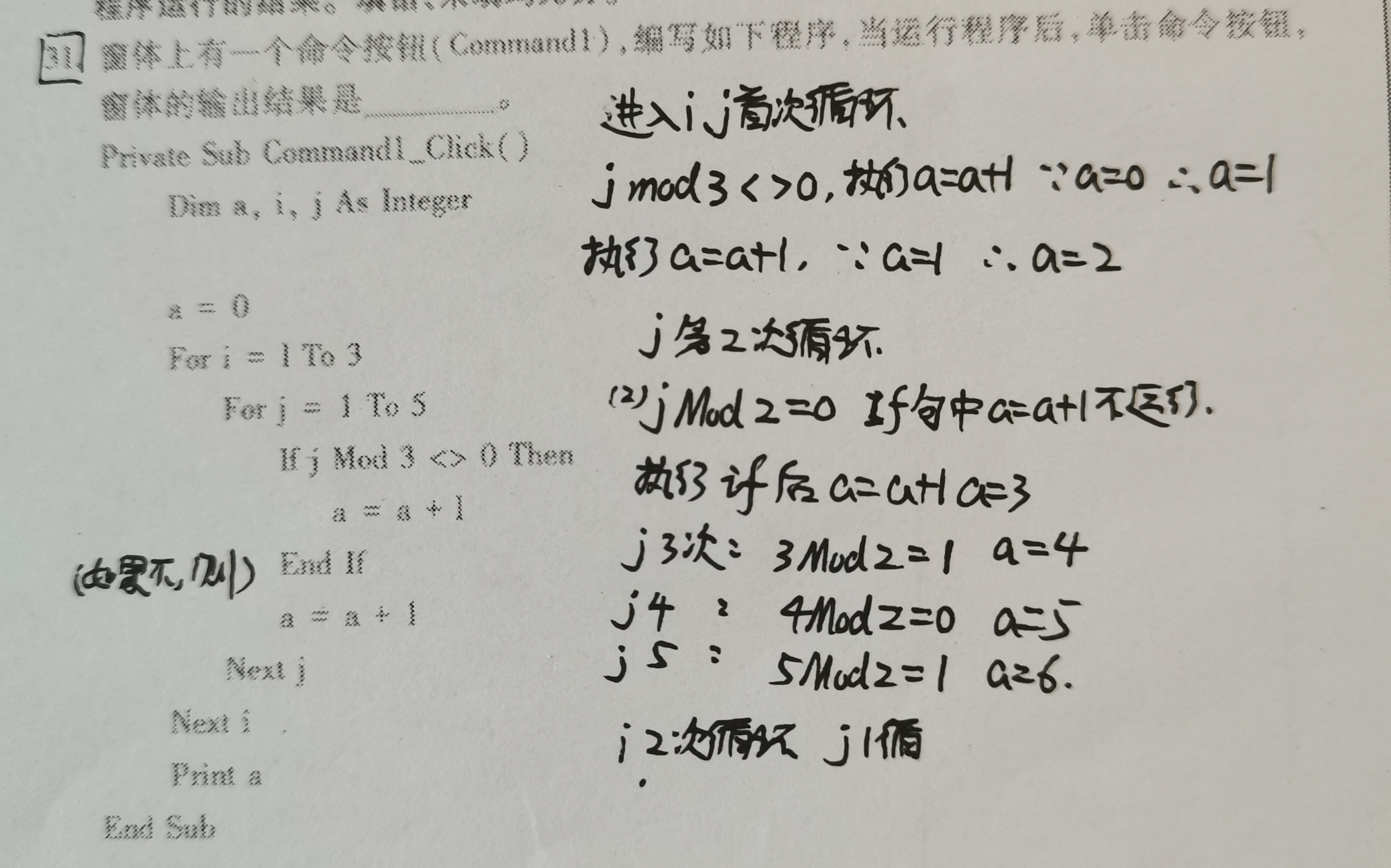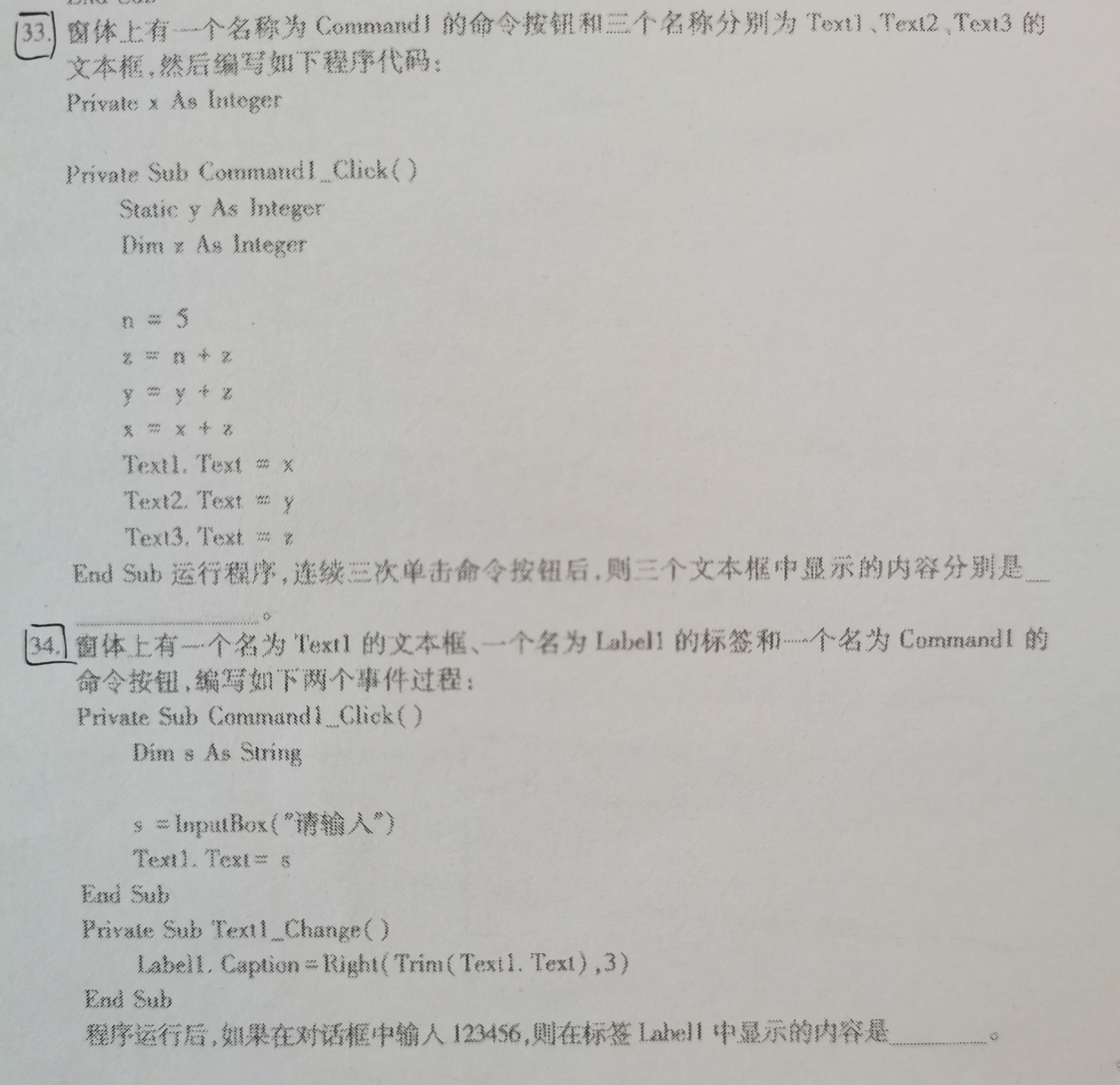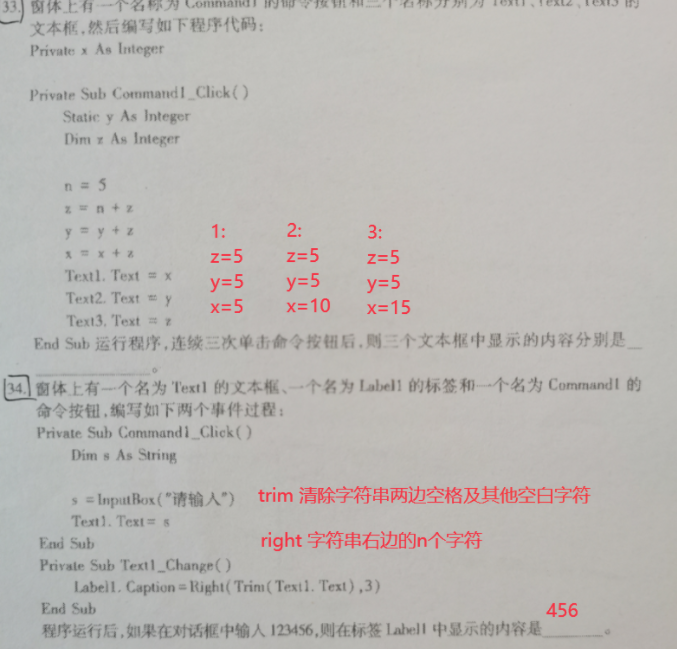2021-07-31 13:20

# 求这几VB题的步骤解释• 点赞
• 收藏

#### 1条回答默认 最新

•浪客 2021-07-31 13:49
已采纳
``````if外面的a=a+1每次循环都会执行，因此，3的倍数是+1，其他都是+2
i=1
j=1 a=2
j=2 a=4
j=3 a=5
j=4 a=7
j=5 a=9
i=2
j=1 a=11
j=2 a=13
j=3 a=14
j=4 a=16
j=5 a=18
i=3
j=1 a=20
j=2 a=22
j=3 a=23
j=4 a=25
j=5 a=27
``````点赞 打赏 评论